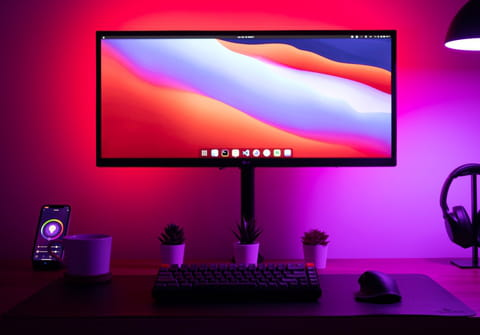# VB6 RGB color: chart, codesA VB6 or (Visual Basic 6) program can be used to find RGB values of a color. Some knowledge of programming concepts and languages such as Visual Basic is required to write a program code in VB6. Basic concepts and knowledge of bit operations, functions, subroutines, etc, are necessary to write programs. A program to find the RGB values of a color in VB6 can be written by using basic bit operations. With binary operations and Visual Basic, finding the RGB values of a color can be accomplished in

To use a VB6 to find the RGB values of a color, use these values:

```Dim R as integer
Dim G as integer
Dim B as integer
Sub FindRGB(Col As Long)
R = &HFF& And Col
G = (&HFF00& And Col ) \ 256
B = (&HFF0000 And Col ) \ 65536
End Sub  ```

Note: Here is a link to more than 500 color descriptions.

Any more programming questions? check out our forum!
Subject Replies